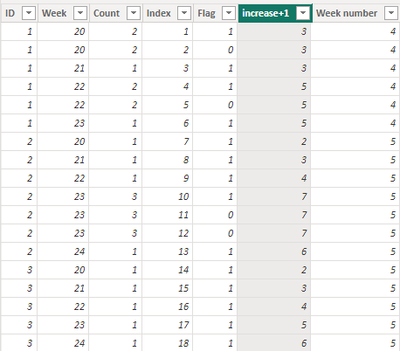cancel
Showing results for
Did you mean:Frequent Visitor

## Frequência de compra semanal

Olá,

Estou tentando criar um código para calcular a semana que os clientes compraram.
Usei o DAX:

Frequencia Compra = DISTINCTCOUNT(TB_FATURAMENTO[N NOTA]) para calcular a frequencia de compra.
Porém preciso de uma fórmula que a cada semana que o cliente comprar acrescente uma compra, independente de quantas vezes ele comprou na semana.

Pode me ajudar?
2 ACCEPTED SOLUTIONSSuper User

Purchase Frequency = Countrows(Summarize(TB_FATURAAMENTO, TB_FATURAAMENTO[Year Week], TB_FATURAAMENTO [N NOTE] ) )Community Support

I created some data:Here are the steps you can follow：

1. In Power Query -- Add Column – Index Column – From 1.2. Create calculated column.

``````Count =
COUNTX(
FILTER(ALL('Table'),
'Table'[ID]=EARLIER('Table'[ID])&&'Table'[Week]=EARLIER('Table'[Week])),[Week number])``````
``````Flag =
var _min=
MINX(FILTER(ALL('Table'),'Table'[ID]=EARLIER('Table'[ID])&&'Table'[Week]=EARLIER('Table'[Week])),[Index])
return
IF(
_min=[Index],1,0)``````
``````increase+1 =
[Count] +
SUMX(FILTER(ALL('Table'),'Table'[ID]=EARLIER('Table'[ID])&&'Table'[Index]<=EARLIER('Table'[Index])),[Flag])``````
``````Week number =
CALCULATE(DISTINCTCOUNT('Table'[Week]),
FILTER(ALL('Table'),'Table'[ID]=EARLIER('Table'[ID])))``````

3. Result:If it doesn't meet your expectations，Can you share sample data and sample output in table format? Or a sample pbix after removing sensitive data. We can better understand the problem and help you

Best Regards,

Liu Yang

If this post helps, then please consider Accept it as the solution to help the other members find it more quickly

2 REPLIES 2Community Support

I created some data:Here are the steps you can follow：

1. In Power Query -- Add Column – Index Column – From 1.2. Create calculated column.

``````Count =
COUNTX(
FILTER(ALL('Table'),
'Table'[ID]=EARLIER('Table'[ID])&&'Table'[Week]=EARLIER('Table'[Week])),[Week number])``````
``````Flag =
var _min=
MINX(FILTER(ALL('Table'),'Table'[ID]=EARLIER('Table'[ID])&&'Table'[Week]=EARLIER('Table'[Week])),[Index])
return
IF(
_min=[Index],1,0)``````
``````increase+1 =
[Count] +
SUMX(FILTER(ALL('Table'),'Table'[ID]=EARLIER('Table'[ID])&&'Table'[Index]<=EARLIER('Table'[Index])),[Flag])``````
``````Week number =
CALCULATE(DISTINCTCOUNT('Table'[Week]),
FILTER(ALL('Table'),'Table'[ID]=EARLIER('Table'[ID])))``````

3. Result:If it doesn't meet your expectations，Can you share sample data and sample output in table format? Or a sample pbix after removing sensitive data. We can better understand the problem and help you

Best Regards,

Liu Yang

If this post helps, then please consider Accept it as the solution to help the other members find it more quicklySuper User